Function Repository Resource:

# FindRootPlot

Visualize the function evaluations done by FindRoot

Contributed by: Wolfram Staff (with additional contributions by Jan Mangaldan)
 ResourceFunction["FindRootPlot"][f,{x,xst}] plots the steps and the points at which the function f and any of its derivatives are evaluated in FindRoot[f,{x,xst}], superimposed on a plot of f versus x. ResourceFunction["FindRootPlot"][{f1,f2},{{x,xst},{y,yst}}] plots the steps and the points at which the pair of functions and their derivatives are evaluated, superimposed on a contour plot of the merit function. ResourceFunction["FindRootPlot"][f,range,property] returns the specified property.

## Details

To simplify processing and reduce possible confusion about the function f, ResourceFunction["FindRootPlot"] does not accept equations; it finds a root of f=0.
In the case of two functions and variables, the merit function is the sum of squares of the pair.
The argument property can be any of the following:
 "Result" the result of FindRoot "Statistics" an association showing the number of steps and evaluations of the function and its derivatives "Plot" the plot of the function and the evaluation points All association containing "Result", "Statistics" and "Plot"
ResourceFunction["FindRootPlot"] takes the same options as FindRoot and Plot (in the one-dimensional case) or ContourPlot (in the two-dimensional case), with the following addition:
 "EvaluationStyles" Automatic styles used for the evaluation points
The "EvaluationStyles" option takes an association whose values correspond to the styles used for the evaluation points in the plot.
With the setting , ResourceFunction["FindRootPlot"] shows a legend for the evaluation points.

## Examples

### Basic Examples (2)

Show the steps and function evaluations used in finding the root of cos(x)=0:

 In:=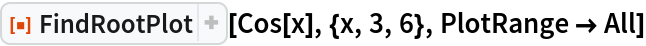Out=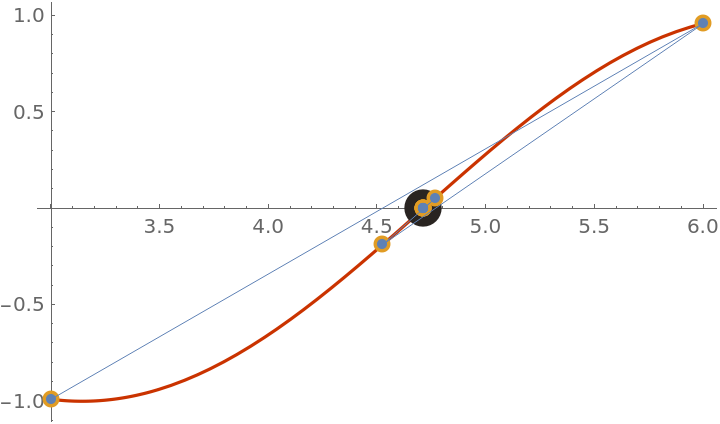Show the steps and function evaluations used in finding the root of the Rosenbrock problem 1-x=0,10(y-x2)=0:

 In:=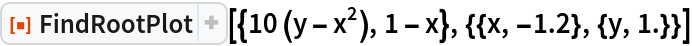Out=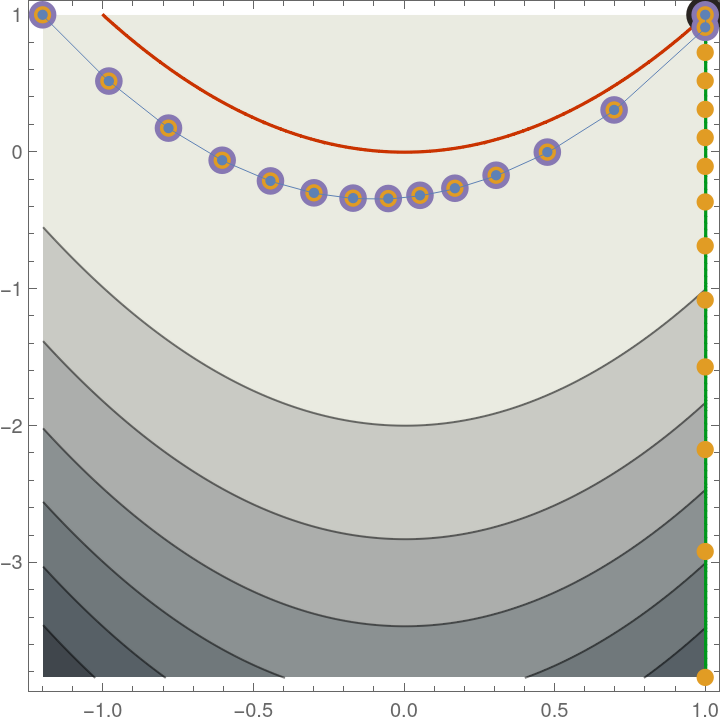### Scope (2)

Use PlotLegends to display a legend for the evaluation points:

 In:=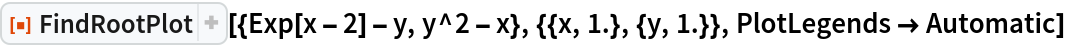Out=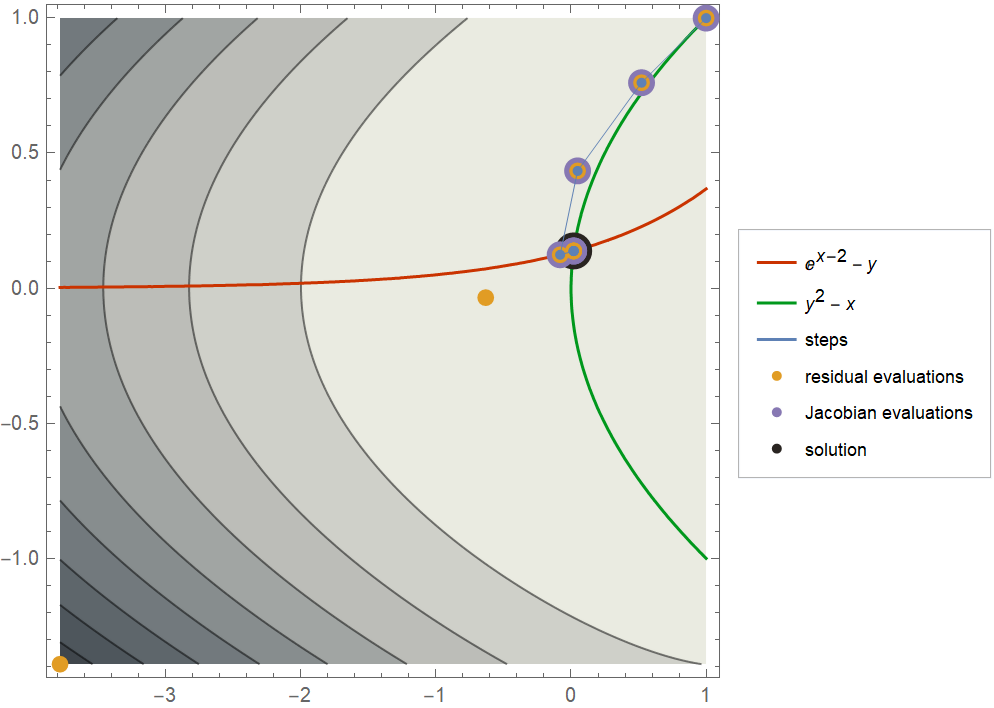Use a different rootfinding method from the default:

 In:=Out=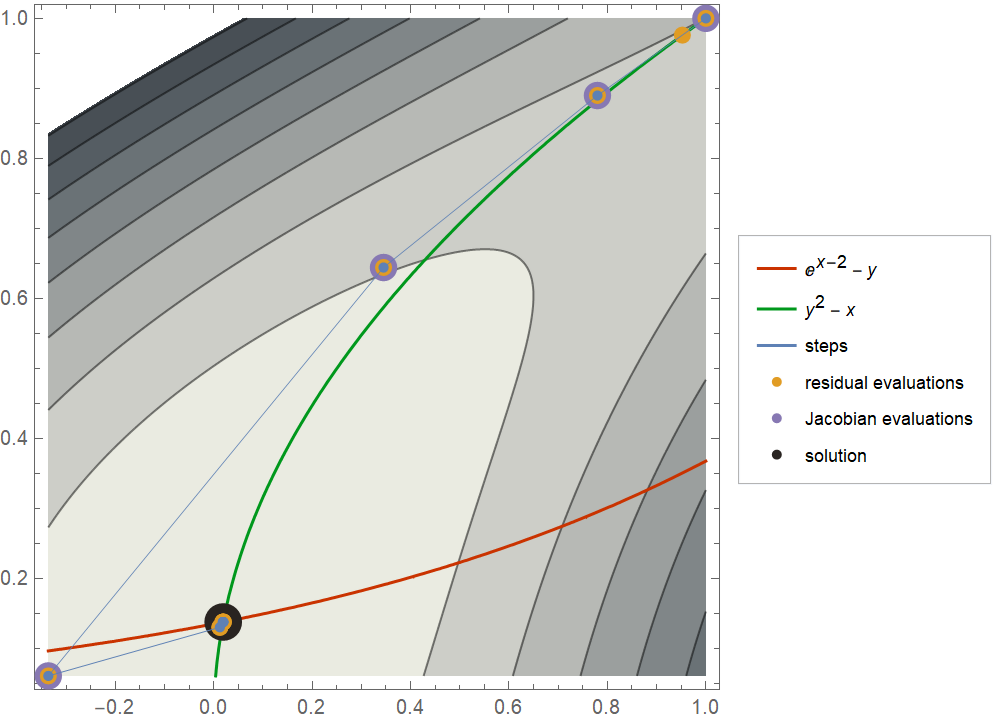Return the statistics of the evaluations done by FindRoot:

 In:=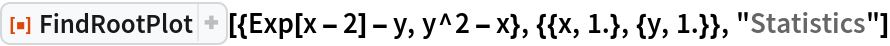Out=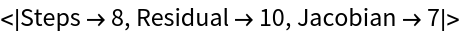Return all properties:

 In:=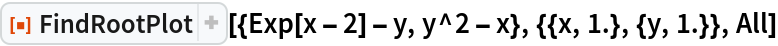Out=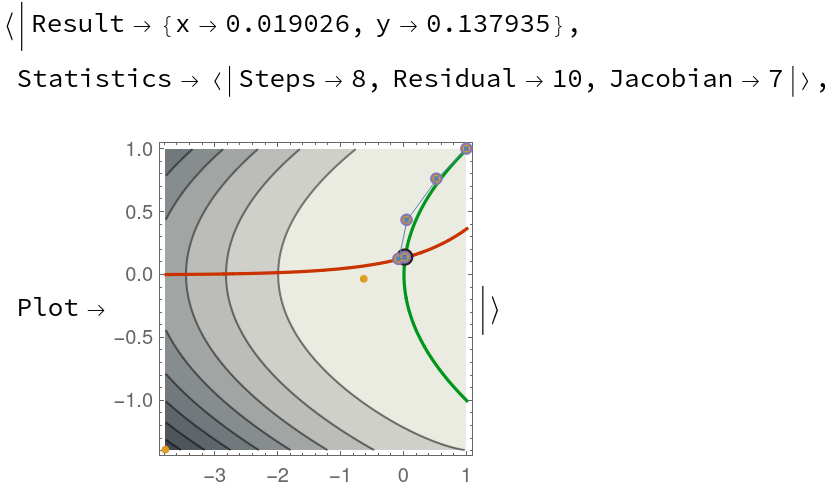### Options (6)

#### ColorFunction (1)

Use a different ColorFunction setting:

 In:=Out=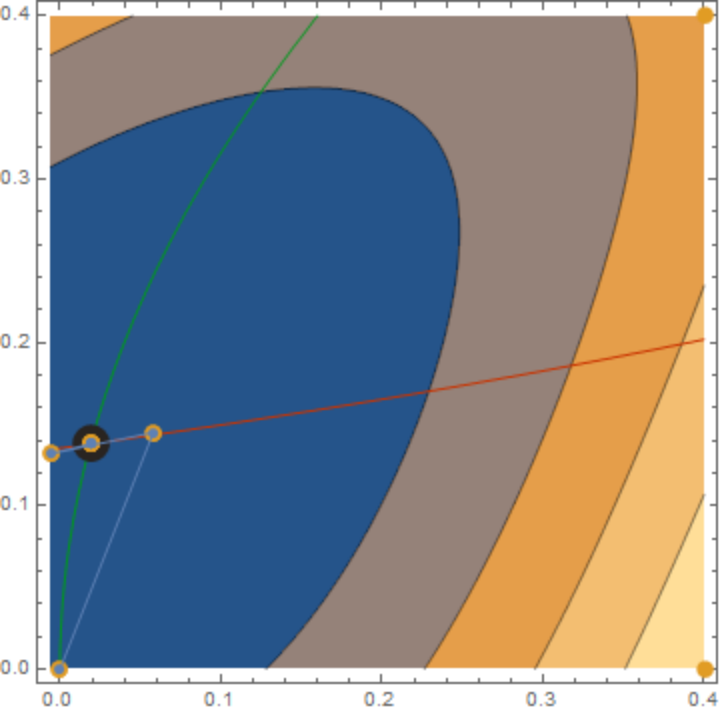#### EvaluationStyles (2)

Use "EvaluationStyles" to change the style used for the evaluation points:

 In:=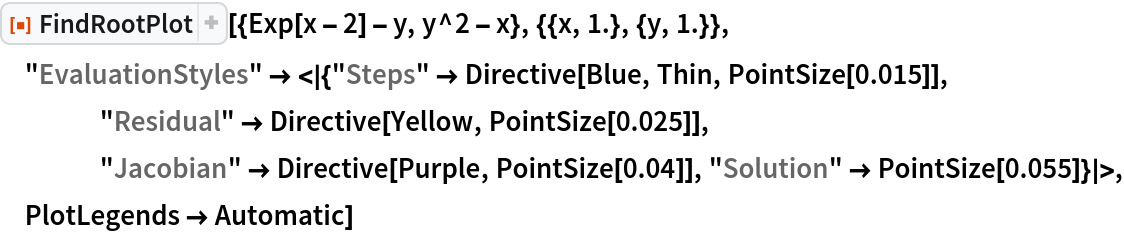Out=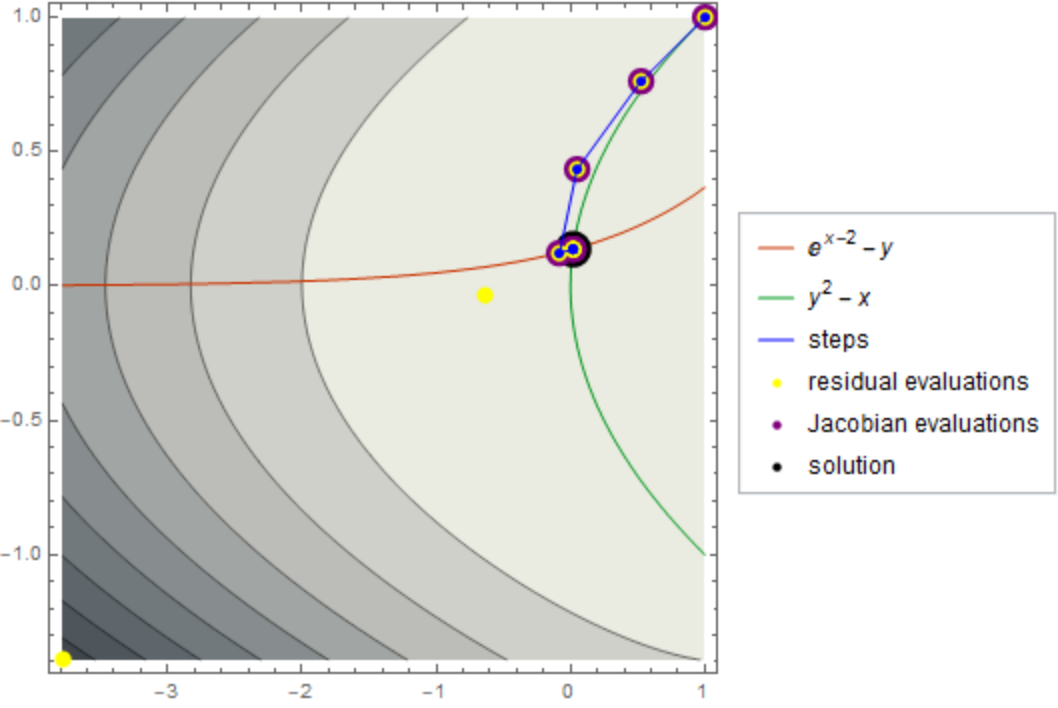Default styles are used if a particular style is left unspecified:

 In:=Out=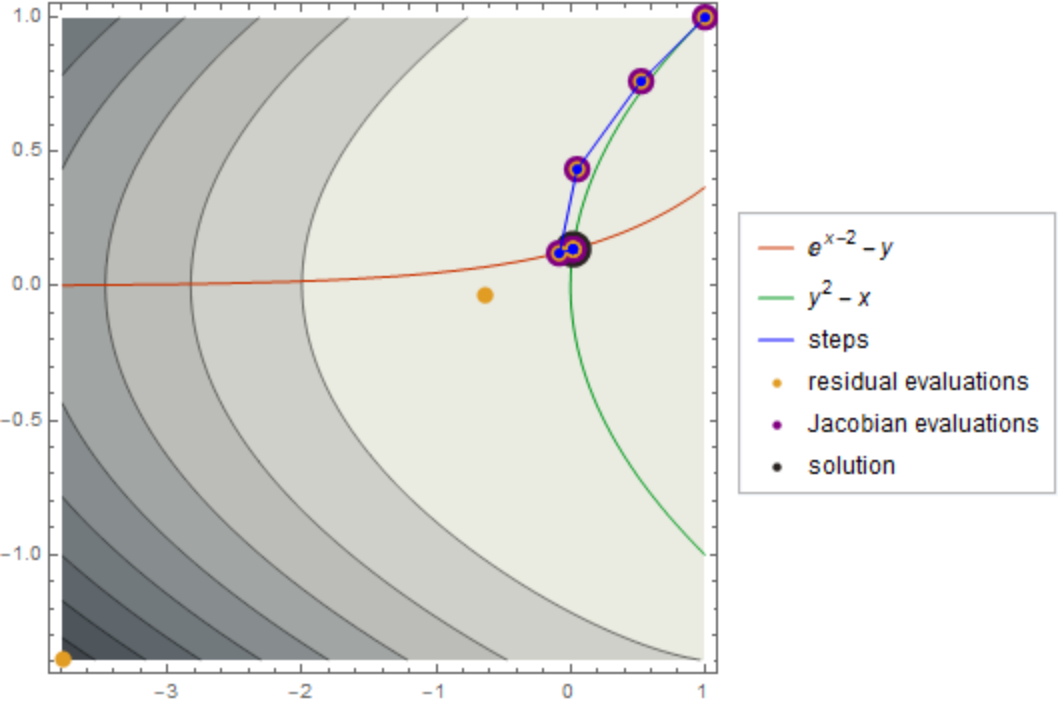#### Method (1)

Compare different rootfinding methods:

 In:=Out=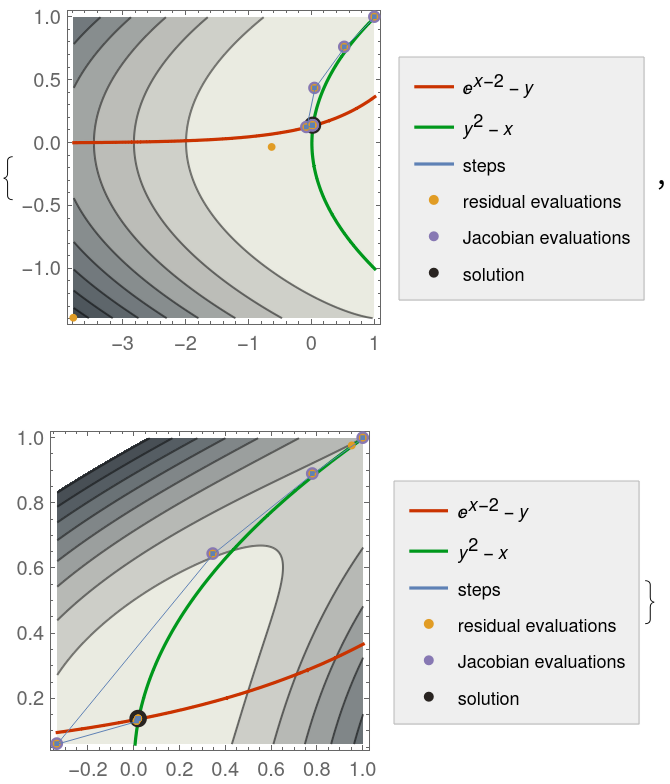#### PlotLegends (1)

Show a legend for the evaluation points:

 In:=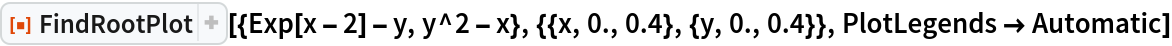Out=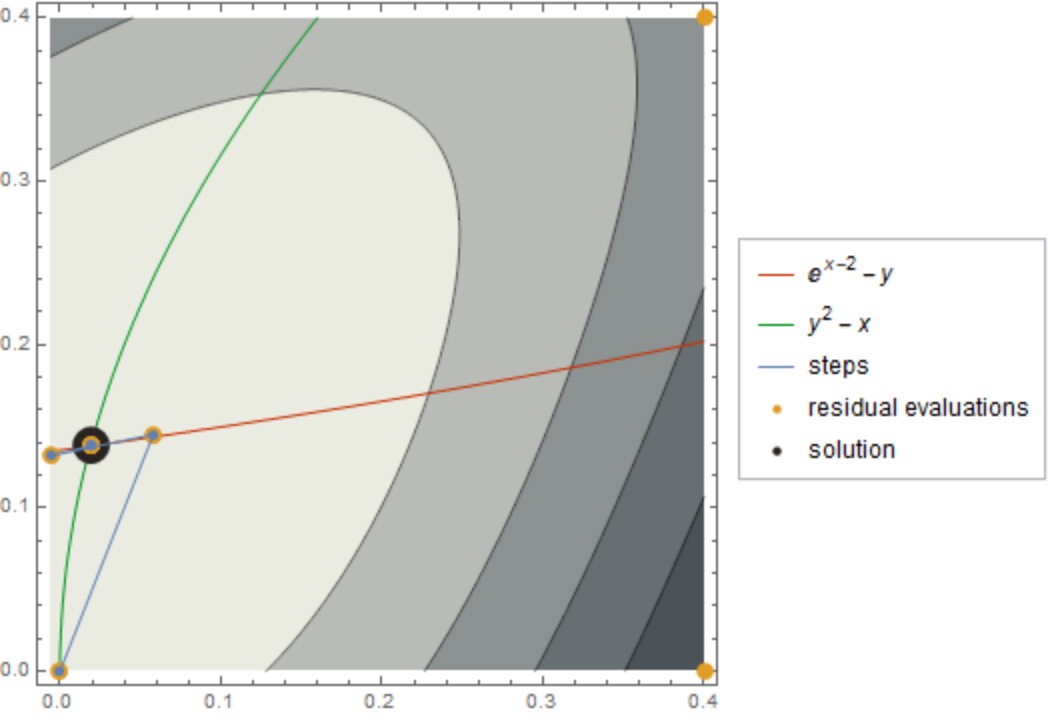#### PlotStyle (1)

Change the style used for the functions:

 In:=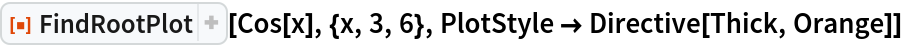Out=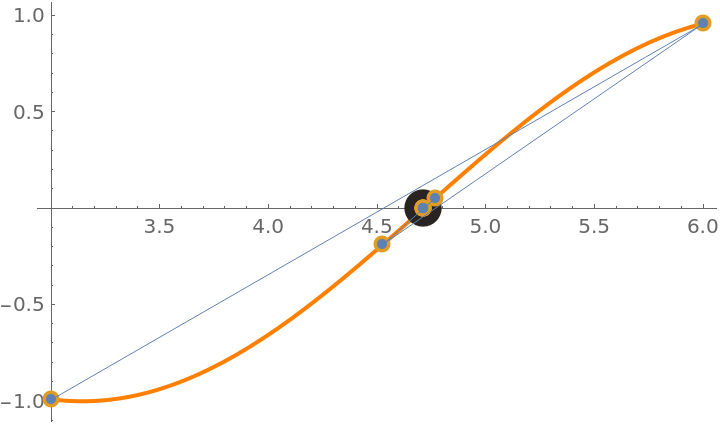In:=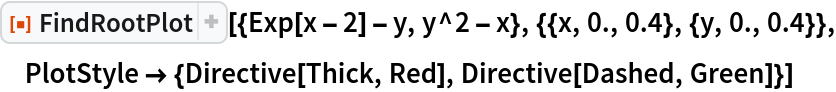Out=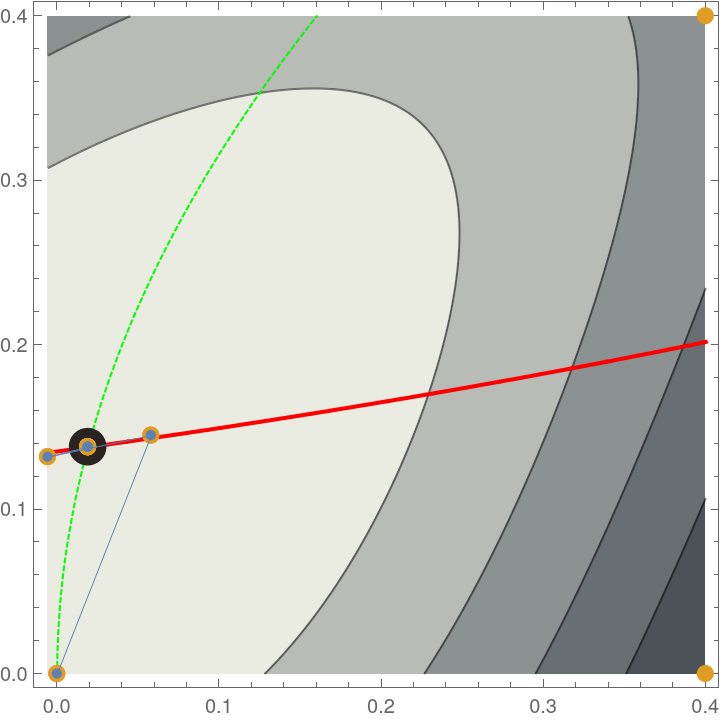## Version History

• 1.0.0 – 16 February 2021